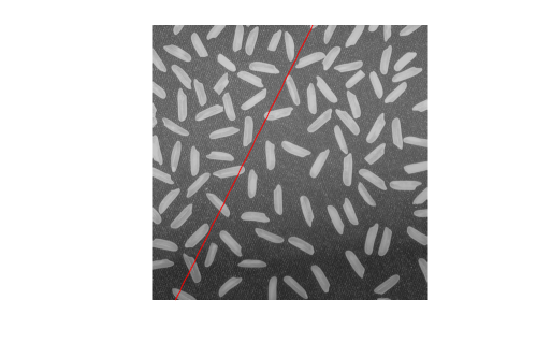Documentation

lineToBorderPoints

Intersection points of lines in image and image border

Description

example

points = lineToBorderPoints(lines,imageSize) computes the intersection points between one or more lines in an image with the image border.

Examples

collapse all

Load and display an image.

figure;
imshow(I);
hold on;Define a line with the equation, 2 * x + y - 300 = 0.

aLine = [2,1,-300];

Compute the intersection points of the line and the image border.

points = lineToBorderPoints(aLine,size(I))
points = 1×4

149.7500    0.5000   21.7500  256.5000

line(points([1,3]),points([2,4]));Input Arguments

collapse all

Line matrix, specified as an M-by-3 matrix, where each row must be in the format, [A,B,C]. This matrix corresponds to the definition of the line:

 A * x + B * y + C = 0.
M represents the number of lines.

lines must be double or single.

Image size, specified as a row vector in the format returned by the size function.

Output Arguments

collapse all

Intersection points, returned as an M-by-4 matrix. The function returns the matrix in the format of [x1, y1, x2, y2]. In this matrix, [x1 y1] and [x2 y2] are the two intersection points. When a line in the image and the image border do not intersect, the function returns [-1,-1,-1,-1].